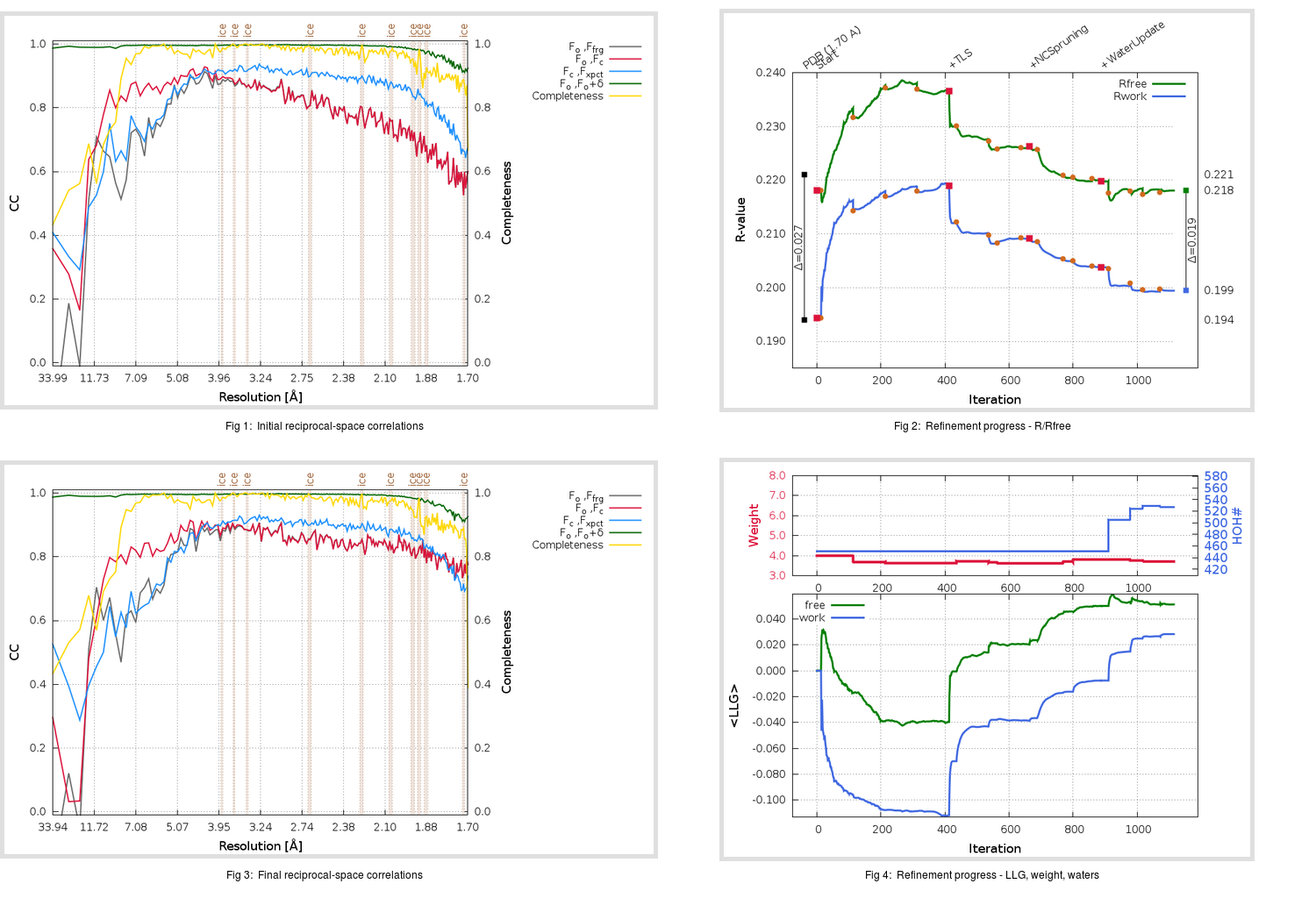Content:

```    Diffraction limits & principal axes of ellipsoid fitted to diffraction cut-off surface:
1.690         0.7502   0.0000   0.6612       0.772 a* + 0.636 c*
1.682         0.0000   1.0000   0.0000       b*
1.668        -0.6612   0.0000   0.7502      -0.502 a* + 0.865 c*
```

## Deposited

` `
 Date deposited Date data collection Resolution R, Rfree 20060721 20051218 1.70 0.1940 0.2210

Molprobity (CCP4 7.0 version) summary:

```Ramachandran outliers =   0.33 %
favored =  97.70 %
Rotamer outliers      =   1.71 %
C-beta deviations     =     0
Clashscore            =   7.67
RMS(bonds)            =   0.0100
RMS(angles)           =   1.65
MolProbity score      =   1.66
Resolution            =   1.70
R-work                =   0.1940
R-free                =   0.2210
```

```Number of waters      =   451

<B> (all atoms) =   32.78 ( sd =   12.70 ) for       5193 non-hydrogen atoms
<B>   (protein) =   32.05 ( sd =   12.79 ) for       4742 non-hydrogen atoms
<B>     (water) =   40.50 ( sd =    8.34 ) for        451 non-hydrogen atoms
<B>    (others) =    0.00 ( sd =    0.00 ) for          0 non-hydrogen atoms

B min/max       (all non-hydrogen atoms) =   12.90 /   89.28
B min/max   (protein non-hydrogen atoms) =   12.90 /   89.28
B min/max     (water non-hydrogen atoms) =   18.72 /   61.08
B min/max     (other non-hydrogen atoms) =    0.00 /    0.00
```

## BUSTER (re-)refinement

` `

Molprobity (CCP4 7.0 version) summary:

```Ramachandran outliers =   0.16 %
favored =  97.70 %
Rotamer outliers      =   2.66 %
C-beta deviations     =     0
Clashscore            =   3.94
RMS(bonds)            =   0.0111
RMS(angles)           =   1.54
MolProbity score      =   1.57
Resolution            =   1.70
R-work                =   0.1994
R-free                =   0.2181
```

```Number of waters      =   527

<B> (all atoms) =   30.69 ( sd =   11.56 ) for       5269 non-hydrogen atoms
<B>   (protein) =   29.53 ( sd =   11.05 ) for       4742 non-hydrogen atoms
<B>     (water) =   41.13 ( sd =   10.77 ) for        527 non-hydrogen atoms
<B>    (others) =    0.00 ( sd =    0.00 ) for          0 non-hydrogen atoms

B min/max       (all non-hydrogen atoms) =   14.50 /   90.83
B min/max   (protein non-hydrogen atoms) =   14.50 /   88.26
B min/max     (water non-hydrogen atoms) =   17.61 /   90.83
B min/max     (other non-hydrogen atoms) =    0.00 /    0.00
```

Refinement progression:Results:

` `
 File Remark 2DUC_aB_refine.01_04_refine.pdb.gz exact refinement commands are in header 2DUC_aB_refine.01_04_refine.mtz.gz including original deposited data and several re-refinement map coefficients 2DUC_aB_refine.01_04_BUSTER_model.cif.gz including any non-standard compound restraints 2DUC_aB_refine.01_04_BUSTER_refln.cif.gz